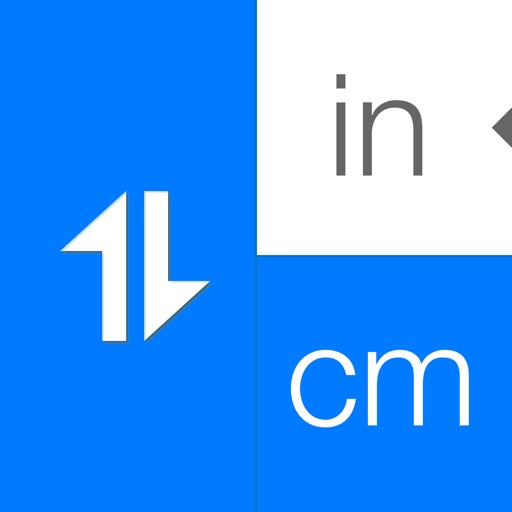## Perfect for your studies or for quick conversions on the go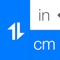# Advanced Unit Converter for Science & Engineering

by 上海宇凌信息科技有限公司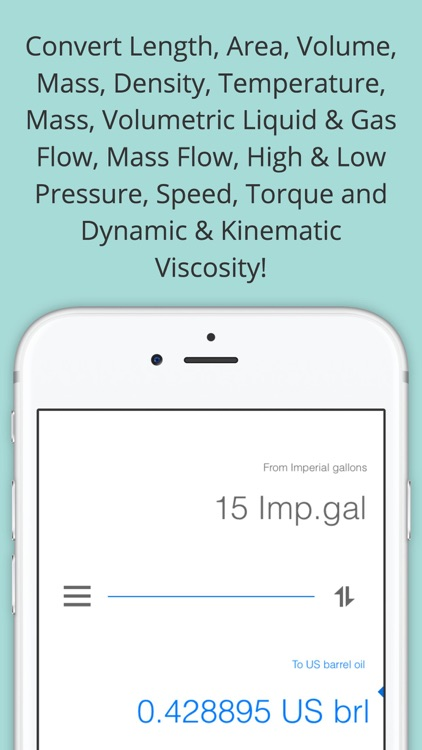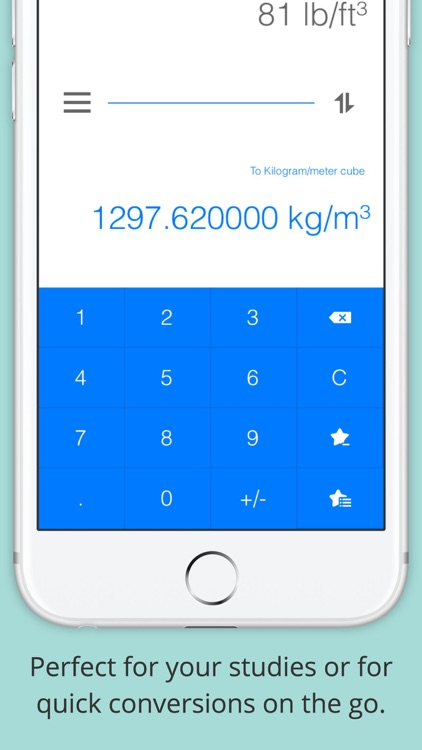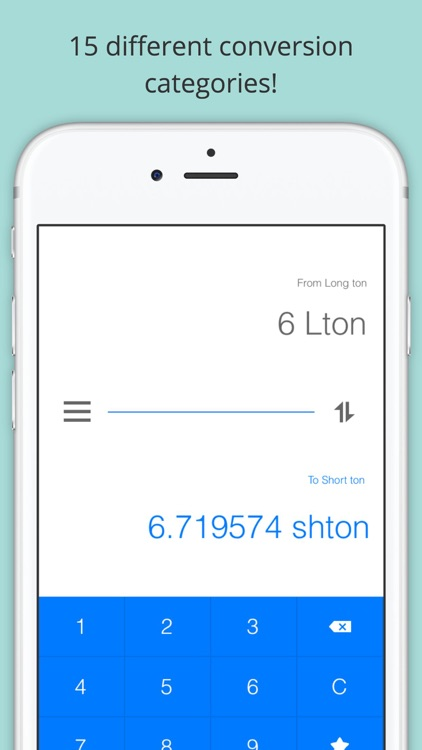Perfect for your studies or for quick conversions on the go.### App Details

Version
1.1.0
Rating
NA
Size
1Mb
Genre
Utilities Education
Last updated
March 10, 2017
Release date
March 8, 2014

### App Store Description

Perfect for your studies or for quick conversions on the go.

15 different conversion categories are included:
- Length: millimeters (mm), centimeters (cm), meters (m), kilometers (km), inches (in), feet (ft), yards (yd), miles (mi)
- Area: mm^2, cm^2, m^2, in^2, ft^2, yd^2
- Volume: cm^3, m^3, liter, in^3, ft^3, US gallons, Imperial gallons, US barrel oil
- Mass: grams (g), kilograms (kg), metric tonnes (t), Short tons (shton), Long tons (lton), pounds (lb), ounces (oz)
- Density: gram per milliliter (g/ml), kilogram per cubic metre (kg/m^3), pound per cubic foot (lb/ft^3), pound per cubic inch (lb/in^3)
- Temperature: degrees Celcius, Fahrenheit and Kelvin
- Volumetric Liquid Flow: liter per second (L/sec), litre per minute (L/min), cubic meter per hour (m^3/hr), cubic foot per minute (ft^3/min), cubic foot per hour (ft^3/hr), US gallons per minute (gal/min), US barrel oil per day (US brl/day)
- Volumetric Gas Flow: normal cubic meter per hour (Nm^3/hr), standard cubic feet per hour (scfh), standard cubic feet per min (scfm)
- Mass Flow: kilogram per hour (kg/h), pounds per hour (lb/h), kilogram per second (kg/s), ton per hour (t/h)
- High Pressure: bar, pound per square inch (psi), kilopascal (kPa), megapascal (MPa), kilogram-force per square centimetre (kgf/cm^2), millimeter of mercury (mm Hg), atmospheres (atm)
- Low Pressure: meter of water column (mH2O), foot of water column (ftH2O), centimeter of mercury (cmHg), inches of mercury (inHg), incher of water column (inH2O), Pascal (Pa)
- Speed: meter per second (m/s), meter per minute (m/min), kilometer per hour (km/h), feet per second (ft/s), feet per minute (ft/min), miles per hour (mi/h)
- Torque: Newton per meter (Nm), kilogram-force per meter (kgfm), foot per pound (ftlb), inche per pound (inlb)
- Dynamic Viscosity: centipoise (cp), poise, pound per foot second (lb/(ft·s))
- Kinematic Viscosity: centistoke (cs), stoke (st), square foot per second (ft^2/s), square meter per second (m^2/s)

Advanced Unit Converter will helps you switch from square meters to square feet, from degrees Celsius to degrees Fahrenheit, from liters to gallons. Easily convert from imperial to metric units and vice versa and much much, more!

With its eye-catching HD graphics, Advanced Unit Converter has been designed from the ground up with simplicity in mind. Intuitive, friendly, with a clean user interface but powerful enough for the most complex conversions.

Disclaimer:
AppAdvice does not own this application and only provides images and links contained in the iTunes Search API, to help our users find the best apps to download. If you are the developer of this app and would like your information removed, please send a request to [email protected] and your information will be removed.